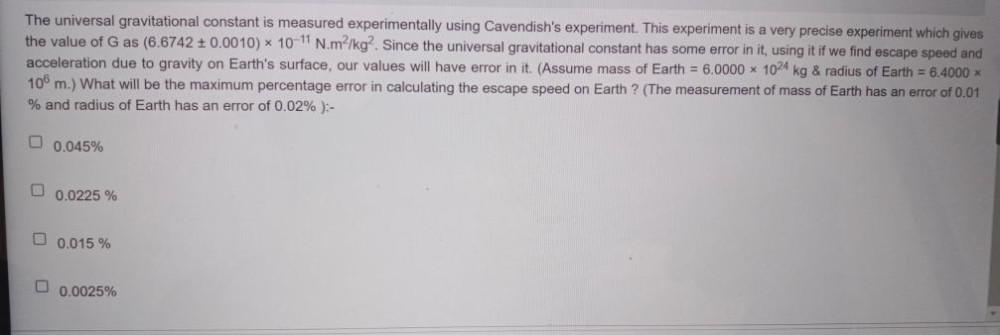Question:

# The universal gravitational constant is measured experimentally using Cavendish's experiment. This experiment is a very preciseThe universal gravitational constant is measured experimentally using Cavendish's experiment. This experiment is a very precise experiment which gives the value of G as (6.6742 + 0.0010) * 10-11 N.m²/kg. Since the universal gravitational constant has some error in it, using it if we find escape speed and acceleration due to gravity on Earth's surface, our values will have error in it. (Assume mass of Earth = 6.0000 x 1024 kg & radius of Earth = 6.4000 x 106 m.) What will be the maximum percentage error in calculating the escape speed on Earth ? (The measurement of mass of Earth has an error of 0.01 % and radius of Earth has an error of 0.02%):- O 0.045% 0.0225 % 0.015 % 0.0025%